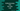# How to find the cube root in python numpy## numpy cbrt method:

cbrt is a numpy method we can use to find the cube root of all elements in an array. In this post, we will learn how to use numpy cbrt with example.

### Definition of cbrt:

numpy cbrt method is defined as below:

``numpy.cbrt(x, /, out=None, *, where=True, casting='same_kind', order='K', dtype=None, subok=True[, signature, extobj]) = <ufunc 'cbrt'>``

Here,

• x is the given array_like value.
• out is the place to put the output array. It is of type ndarray. It is optional. If it is not provided, or if it is None, a freshly allocated array is returned.
• where is a condition that is used to each members of the array. If it is True, it will put the ufunc result to the out array.
• kwargs keyword only arguments.

It returns a ndarray. It is the same shape as the input array.

### Example of cbrt:

Let’s take a look at the example below:

``````import numpy as np

print(np.cbrt([1, 8, 64]))``````

It will print the below output:

``[1. 2. 4.]``

We can also use it with any dimension array:

``````import numpy as np

print(np.cbrt([[1.4, 8.4, 64.8], [2, 5.8, 10.4]]))``````

It will print:

``````[[1.11868894 2.03279271 4.0165977 ]
[1.25992105 1.79670178 2.18278577]]``````